## Filters

Sort by :
Clear All
Q

6. In the given figures below, decide whether is parallel to .(i) In this case. the sum of the interior angle is  thus l is not parallel to m.\ (ii) In this case also l is not parallel to m as the corresponding angle cannot be   (Linear pair will not form). (iii) In this l and m are parallel. This is because the corresponding angle is  and it forms linear pair with   . (iv) The lines are not parallel as the linear pair not form. (Since corresponding angle...

5. In the given figure, the arms of two angles are parallel. If , then find(i) Since side AB is parallel to DG.       Thus :                           (Corresponding angles of parallel arms are equal.)   (ii)     Further side BC is parallel to EF. We have :                             (Corresponding angles of parallel arms are equal.)

4.(ii)  Find the value of in each of the following figures if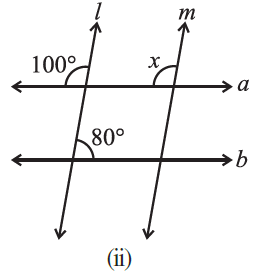The value of x is ,  as these are the corresponding angles.

4.(i) Find the value of in each of the following figures if .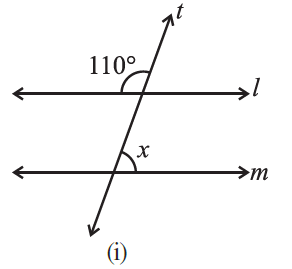The linear pair of the  is :                Thus the value of x is :                                        (Corresponding angles of parallel lines are equal).

3. In the adjoining figure,. Find the unknown angles.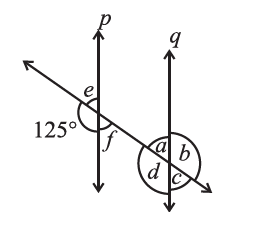The angles can be found using different properties: (a)         (The angles are linear pair) (b)                              (Vertically opposite angle) (c)                                            (Corresponding angle) (d)                             (Vertically opposite angle) (e)              (Vertically opposite angel,  linear pair).

2.  In the adjoining figure, identify

(i) the pairs of corresponding angles.
(ii) the pairs of alternate interior angles.
(iii) the pairs of interior angles on the same side of the transversal.
(iv) the vertically opposite angles.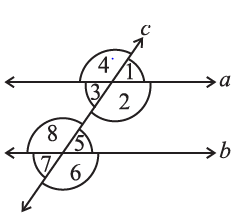(i) Corresponding angles :-    ,  ,  ,   (ii) Alternate interior angles :-    ,  ,   (iii) Alternate angles on the same side of traversal :-   ,   (iv) Vertically opposite angles :-       , ,  ,

1.(iii)  State the property that is used in each of the following statements?

(iii) If  , then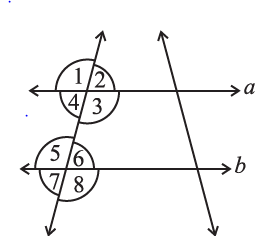The property used here is 'Interior angles on the same side of the transversal are a pair of supplementary angles'.

1.(ii)   State the property that is used in each of the following statements?

(ii) If  , then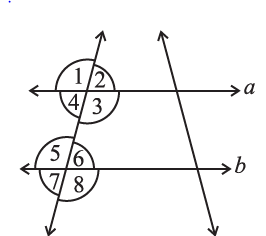The property used here is 'alternate interior angle property'.

1.(i) State the property that is used in each of the following statements?

(i) If  , then .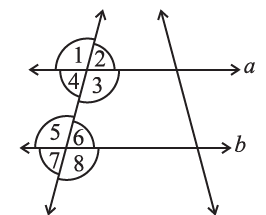The statement  "If  , then   "  is true using the corresponding angles property.
Exams
Articles
Questions